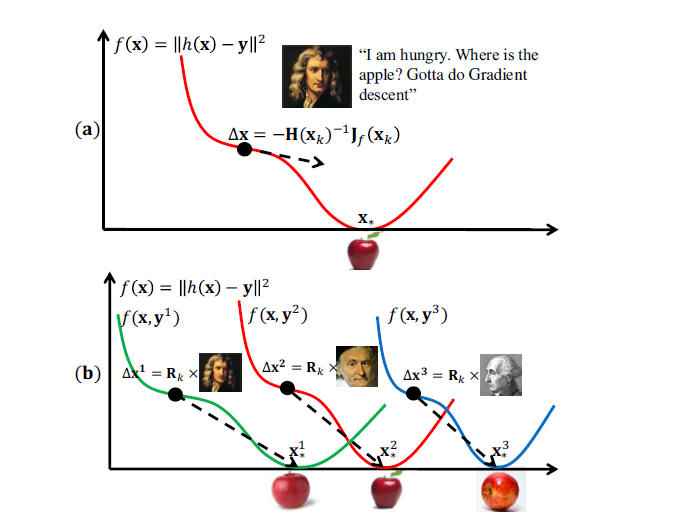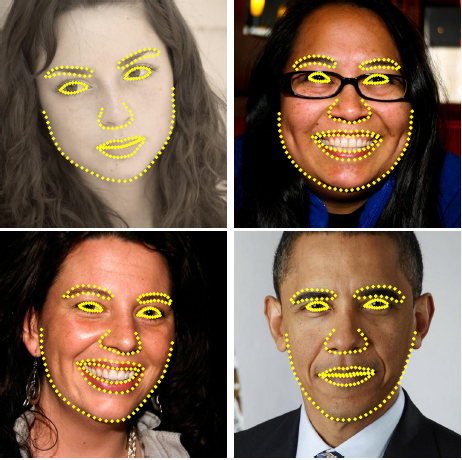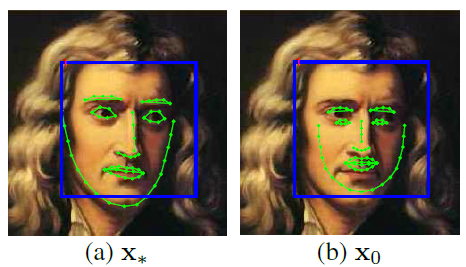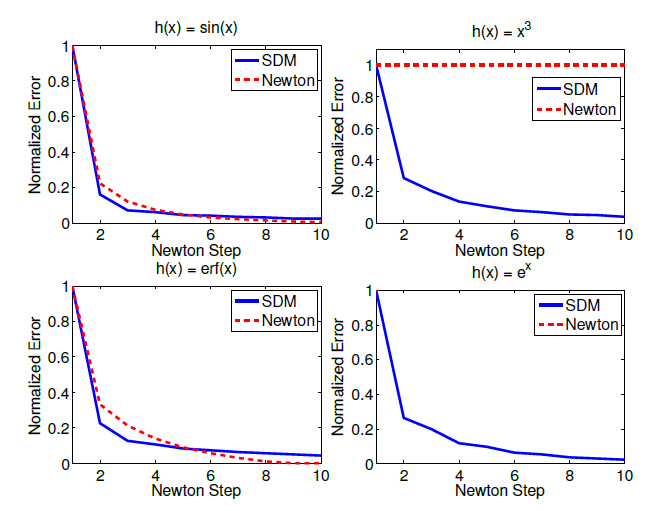## Supervised Descent Method(人脸对齐之SDM论文解析）

Supervised Descent Method(人脸对齐之SDM论文解析）

## 2.从牛顿步说起

xk+1=xkH1(xk)Jf(xk)(1)

1. 如果牛顿步可以收敛，那么它的收敛速度是二次的，收敛速度非常快。
2. 如果初始点在最小点邻域附近，那么它一定可以收敛。

1. Hessian矩阵在极小值附近是局部正定的，但可能不是全局正定的，这就会导致牛顿步并不一定朝向下降的方向。
2. 牛顿步需要函数二次可导。这个要求在实际应用中是一个很强的要求，比如图像处理中经常被使用的SIFT特征，它可以被看成是一个不可导的特征，因此在这种情况下，在我们只能通过数值逼近下降的方向或者是Hessian矩阵，但这种计算代价非常大。
3. 由于Hessian矩阵通常很大，计算它的逆矩阵代价是非常大的，复杂度通常是 O(p3) $O(p^3)$## 3.人脸对齐的几个概念（简单介绍）SDM方法属于基于回归的方法。

## 4.Supervised Descent Method

f(x0+Δx)=||h(d(x0+Δx))ϕ||22(2)f(x0+Δx)=||h(d(x0+Δx))ϕ||22(3)

x0=1NNi=1xi(4) $x_0=\frac1N\sum_{i=1}^Nx_{*i}\tag{4}$

f(x)=f(x0)+Jf(x0)T(xx0)+12(xx0)TH(x0)(xx0)+o(|xx0|2)(5)

f(x)=f(x0)+Jf(x0)T(xx0)+12(xx0)TH(x0)(xx0)(6)

df(x)dx=f(x)(7)

df(x0)dx=0(8)

dJf(x0)T(xx0)dx=Jf(x0)(9)

d12(xx0)TH(x0)(xx0)dx=12[H(x0)+H(x0)T](xx0)=H(x0)(xx0)(10)

f(x)=0+Jf(x0)+H(x0)(xx0)(11)

f(x)=Jf(x0)+H(x0)(xx0)=0(12)

x=x0H1(x0)Jf(x0)(13)

x1=x0H1(x0)Jf(x0)(14)

Δx1=H1(x0)Jf(x0)(15)

xk+1=xk+Δxk(16)

df(g(x))dx=dgT(x)dxdf(g)dg(17)

Jf(x0)=df(x)dx|x=x0=d||h(d(x))ϕ||22dx|x=x0=d(ϕxϕ)Tdx|x=x0d||ϕxϕ||22d(ϕxϕ)|x=x0

(18)

d(ϕxϕ)Tdx|x=x0=dϕTxdx|x=x0=dhT(d(x))dx|x=x0=JTh(x0)(19)

d||ϕxϕ||22d(ϕxϕ)|x=x0=d[(ϕxϕ)T(ϕxϕ)]d(ϕxϕ)|x=x0=2(ϕxϕ)|x=x0=2(ϕ0ϕ)(20)

Jf(x0)=2JTh(x0)(ϕ0ϕ)(21)

x=x0H1(x0)Jf(x0)=x02H1(x0)JTh(x0)(ϕ0ϕ)(22)

Δx1=2H1(x0)JTh(x0)(ϕ0ϕ)(23)

Δx1=2H1(x0)JTh(x0)(ϕ0ϕ)=Δx1=2H1(x0)JTh(x0)ϕ02H1(x0)JTh(x0)ϕ

(24)

R0=2H1(x0)JTh(x0) $R_0=-2H^{-1}(x_0)J^T_h(x_0)$ b0=2H1(x0)JTh(x0)ϕ $b_0=-2H^{-1}(x_0)J^T_h(x_0)\phi_*$,可将上式表示成:
Δx1=R0ϕ0+b0(25)

loss=||Δx1R0ϕ0b0||22(26)SDM方法在更新 x $x$时，就是将更新的增量 Δx $\Delta x$的计算方法进行了改变，由牛顿步的计算Jacobian和Hessian矩阵来得到增量 Δx $\Delta x$，变成了计算 Rk $R_k$ bk $b_k$来得到增量 Δx $\Delta x$,通过推导将每一次的增量 Δx $\Delta x$变成了该次输入的特征 ϕ $\phi$的一次函数，并通过最小二乘直接计算一次函数的系数 Rk $R_k$ bk $b_k$，大大减少了计算量。